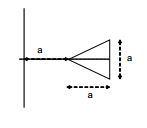# Mutual inductance of a long wire and a triangular loop?

• TheSodesa
In summary, the mutual inductance between a long straight wire and a conducting loop shaped like an isosceles triangle is M = \frac{N_1\phi _1}{I_2} = \frac{N_2\phi _2}{I_1} where B is the magnetic flux density through the loop and A the area of the loop.

#### TheSodesa

I originally posted this to the advanced homework -section, but maybe this isn't advanced enough to warrant being posted there. If a mod sees this, feel free to delete the original on the other forum. I'd do it myself, but I can't.

1. Homework Statement

Calculate the mutual inductance for the long, straight conductor and a conducting loop shaped like an isosceles triangle in the picture below, when a = 10cm.## Homework Equations

Mutual inductance: $M = \frac{N_1\phi _1}{I_2} = \frac{N_2\phi _2}{I_1}$

Magnetic flux: $\phi = BA$, where B is the magnetic flux density through a loop and A the area of the loop.

Magnetic flux density for a long straight wire(Ampere): $B=\mu H = \frac{\mu I}{2 \pi R}$, where R is the orthogonal distance from the wire.

## The Attempt at a Solution

In order to calculate M, I need to find out the total flux through the triangular loop. We already know the expression for B, however dA is a bit of a mystery.

Looking at the picture, if at a distance r from the vertex of the triangle we mark:
\begin{cases}
\text{Height of top half of triangle} = y(r)\\
\text{Distance from the vertex} = r
\end{cases}
Then by similarity:
$$\frac{1/2 a}{a} = \frac{y(r)}{r} \iff y(r) = \frac{r}{2}$$
and if we slice the triangle into small slices with height 2y and width dr:
$$dA=2ydr = r dr$$
Now $$d\phi = d(B(r)A(r)) = B(r)dA(r)$$

Therefore
\begin{array}{ll}
d\phi
&= \frac{\mu I r dr}{2 \pi (a+r)}\\
&= \frac{\mu I r dr}{2r \pi (\frac{a}{r}+1)}\\
&= \frac{\mu I dr}{2 \pi (\frac{a}{r}+1)}\\
&= \frac{\mu I dr}{2 \pi (\frac{a}{r}+1)}\\
&= \frac{\mu I}{2 \pi }\frac{dr}{(\frac{a}{r}+1)}
\end{array}
Looking at this it doesn't seem to be possible to integrate this expression with respect to r from 0 to a. My guess is my derivation of dA is wrong. Any help on that front?

Last edited:
Let's try this again:

$$d\phi = \frac{\mu I}{2 \pi }\frac{dr}{(\frac{a}{r}+1)}$$

Then
$$\phi = \int_{0}^{a}\frac{\mu I}{2 \pi }\frac{dr}{(\frac{a}{r}+1)}$$
$$\phi = \frac{\mu I}{2 \pi } \int_{0}^{a}\frac{dr}{(\frac{a}{r}+1)}$$
$$\phi = \frac{\mu I}{2 \pi } \int_{0}^{a}\frac{r}{(a+r)}dr$$
Now let's do long division on our rational expression (off screen, 'cause duck doing that in LaTeX):
$$\frac{r}{(a+r)} = 1 + \frac{-a}{r+a} = 1 - \frac{a}{r+a}$$
This looks like something we can work with, so let's replace the integrand with our quotient:
$$\phi = \frac{\mu I}{2 \pi } \int_{0}^{a}\frac{r}{(a+r)}dr$$
$$\phi = \frac{\mu I}{2 \pi } \int_{0}^{a}1-\frac{a}{r+a}dr$$
$$\phi = \frac{\mu I}{2 \pi } [r-a \ ln(r+a)]_{0}^{a}$$
$$\phi = \frac{\mu I}{2 \pi } (a-a \ ln(2a) - 0 + a \ ln(a))$$
$$\phi = \frac{\mu aI}{2 \pi } (1-ln(2a) - 0 + ln(a))$$
$$\phi = \frac{\mu aI}{2 \pi } (1+ln(\frac{a}{2a}))$$
Then by substituting this for ##\phi \ in \ M##:
$$M = \frac{\phi}{I} = \frac{\frac{\mu aI}{2 \pi } (1+ln(\frac{1}{2}))}{I}$$
$$M = \frac{\mu a}{2 \pi } (1+ln(\frac{1}{2}))$$
and by plugging in ##a = 10cm## and ##\mu = 4\pi*10^{-7}NA^{-2}## :
$$M = \frac{(4\pi*10^{-7}NA^{-2})(10cm)}{2 \pi } (1+ln(\frac{1}{2})) = 6.13706... × 10^-9 \frac{Nm}{A^2}$$
$$M \approx 6.1 nH$$
Booyah!

•